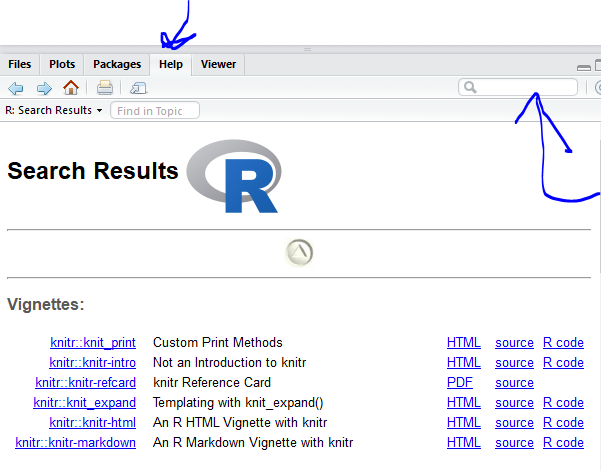## 4.7 Functions and their arguments

Functions are “canned scripts” that automate more complicated sets of commands including operations assignments, etc. Many functions are predefined, or can be made available by importing R packages (more on that later). A function usually gets one or more inputs called arguments. Functions often (but not always) return a value.

A typical example would be the function `round`()`. The input (the argument) must be a number, and the return value (in fact, the output) is that number rounded to the nearest whole number. Executing a function (‘running it’) is called calling the function. You can save the output of a function to an object. The format would look like:

``b <- round(a)``

Here, the value of `a` is given to the `round`()`function, the`round()`function rounds the number, and returns the value which is then assigned to the object`b`.

The return ‘value’ of a function need not be numerical (like that of `sqrt()`), and it also does not need to be a single item: it can be a set of things, or even a dataset. We’ll see that when we read data files into R.

Arguments can be anything, not only numbers or filenames, but also other objects. Exactly what each argument means differs per function, and must be looked up in the documentation (see below). Some functions take arguments which may either be specified by the user, or, if left out, take on a default value: these are called options. Options are typically used to alter the way the function operates, such as whether it ignores ‘bad values,’ or what symbol to use in a plot. However, if you want something specific, you can specify a value of your choice which will be used instead of the default.

`round()` only needs one argument, a number, or object that is storing a numerical value.

``round(percent_vax)``
``##  66``

Here, we’ve called `round()` on our percent_vax object and it returned 66. That’s because the default action of the function is to round to the nearest whole number. If we want more digits we can see how to do that by getting information about the `round` function. We can use `args(round)` or look at the help for this function using `?round`.

``args(round)``
``````## function (x, digits = 0)
## NULL``````
``?round``

We see that if we want a different number of digits, we can type `digits=2` or however many we want.

``round(percent_vax, digits = 2)``
``##  66.37``

If you provide the arguments in the exact same order as they are defined you don’t have to name them:

``round(percent_vax, 2)``
``##  66.37``

And if you do name the arguments, you can switch their order:

``round(digits = 2, x = percent_vax)``
``##  66.37``

It’s good practice to put the non-optional arguments (like the number you’re rounding) first in your function call, and to specify the names of all optional arguments. If you don’t, someone reading your code might have to look up the definition of a function with unfamiliar arguments to understand what you’re doing.

### 4.7.1 Challenge: using functions

Questions

Look at the documentation for the `seq` function. What does `seq` do? Give an example of using `seq` with either the `by` or `length.out` argument.

### 4.7.2 Getting Help

To get help about a particular package or function you can access the help pane in RStudio and type its name in the search box.The `help()` function and `?` help operator in R provide access to the documentation pages for R functions, data sets, and other objects, both for packages in the standard R distribution and for contributed packages. To do so type as follows

``````help({function})
help(package = {package name})

?{function}
?"{package name}"``````